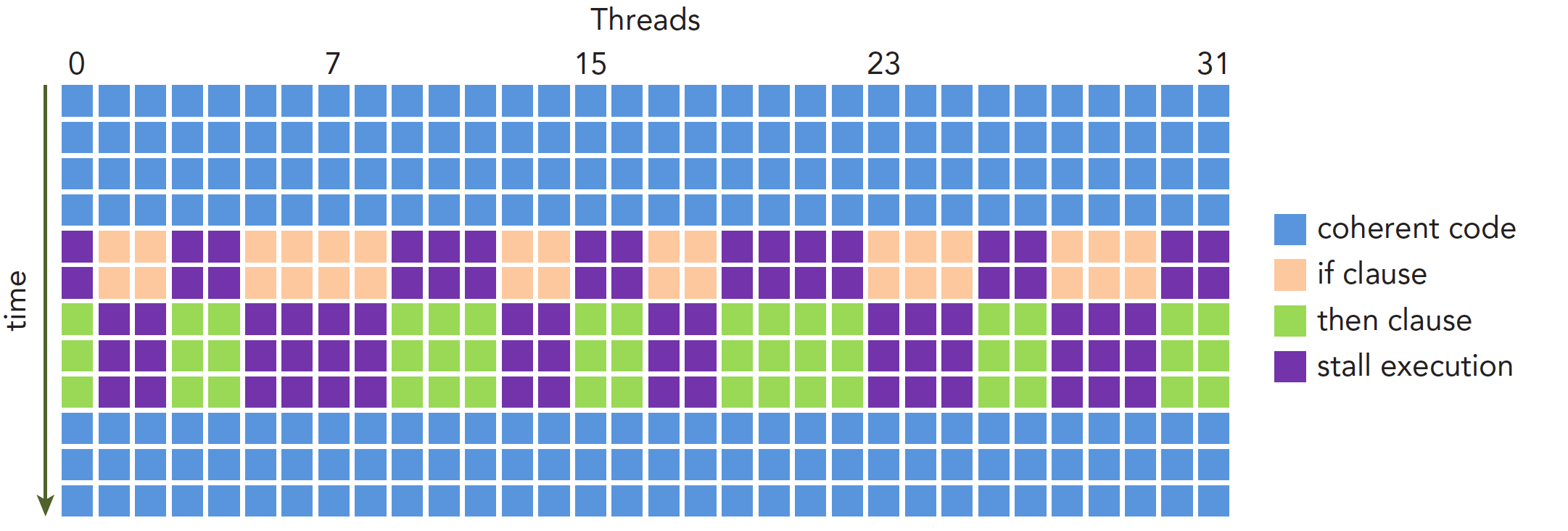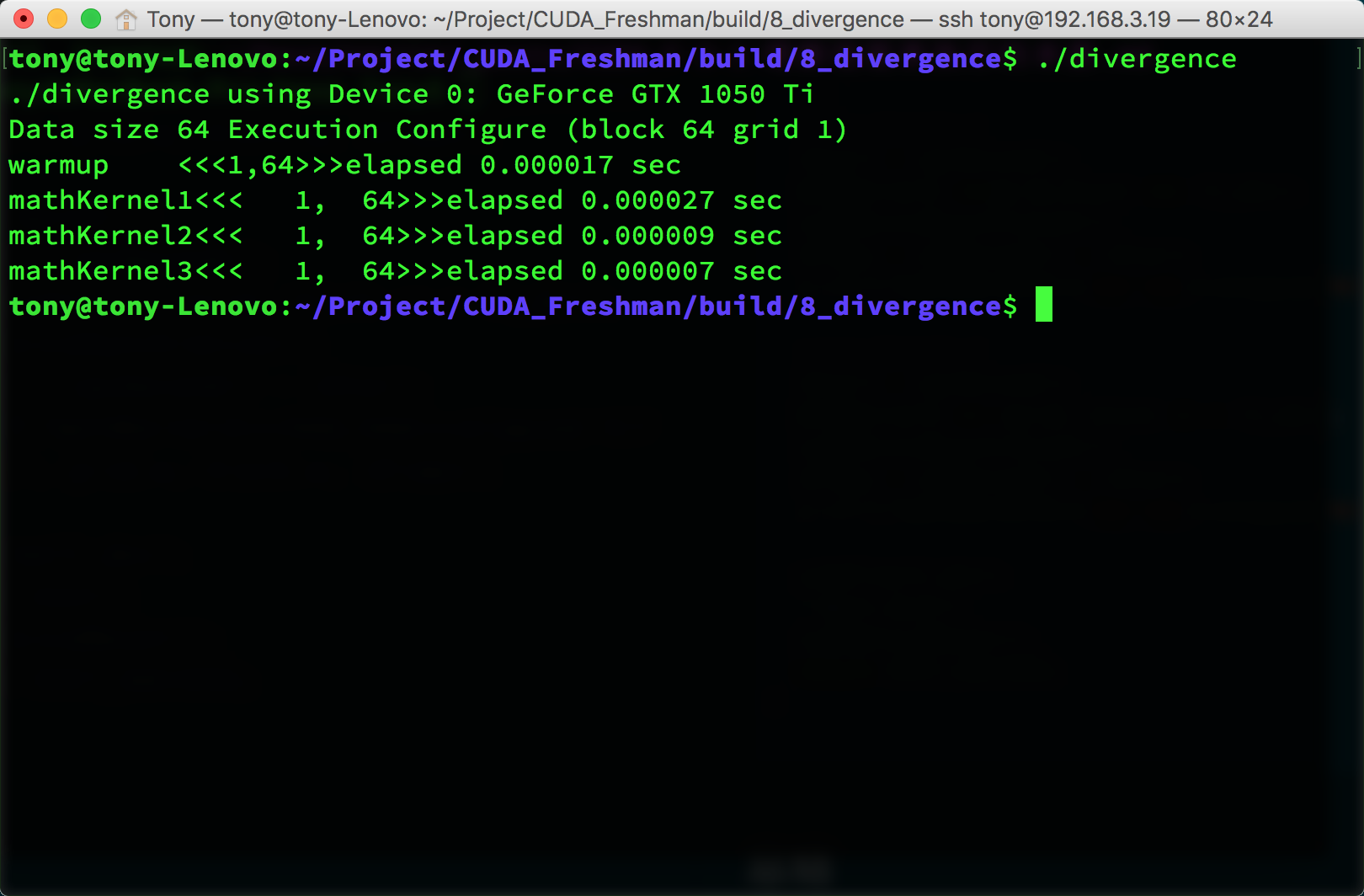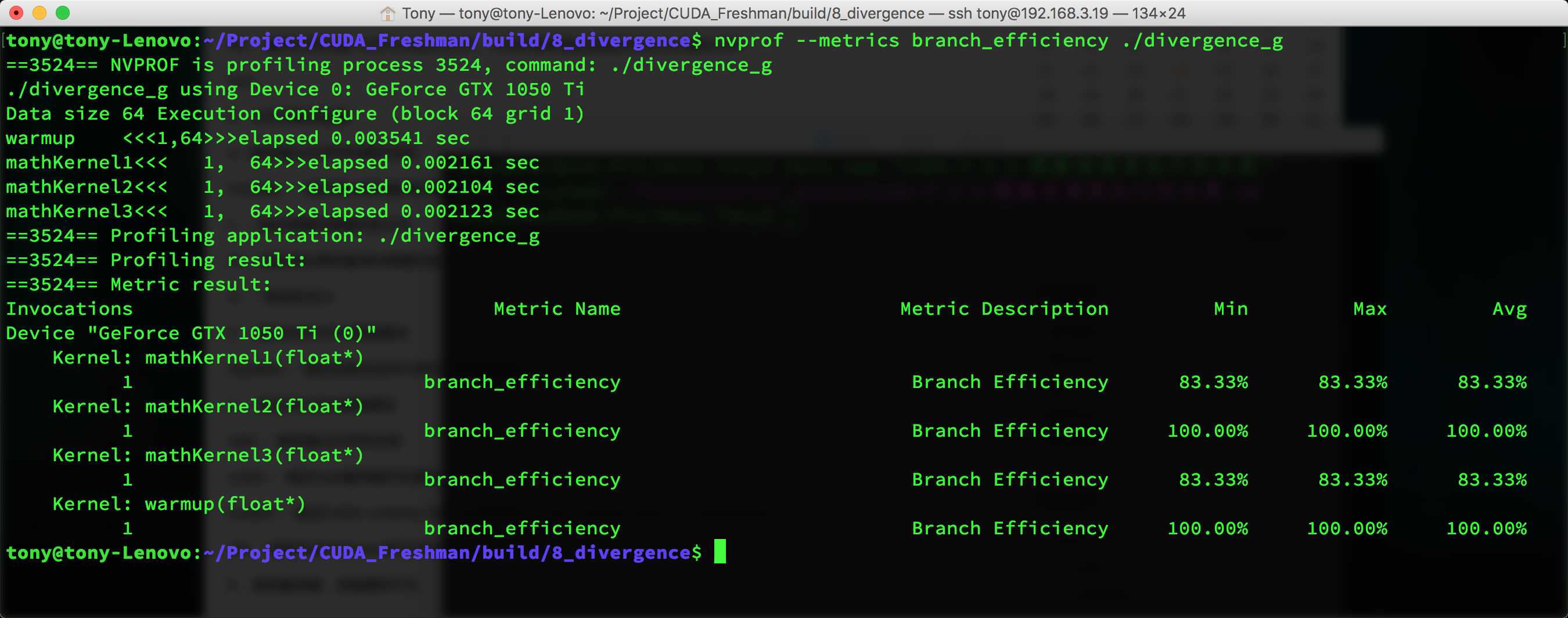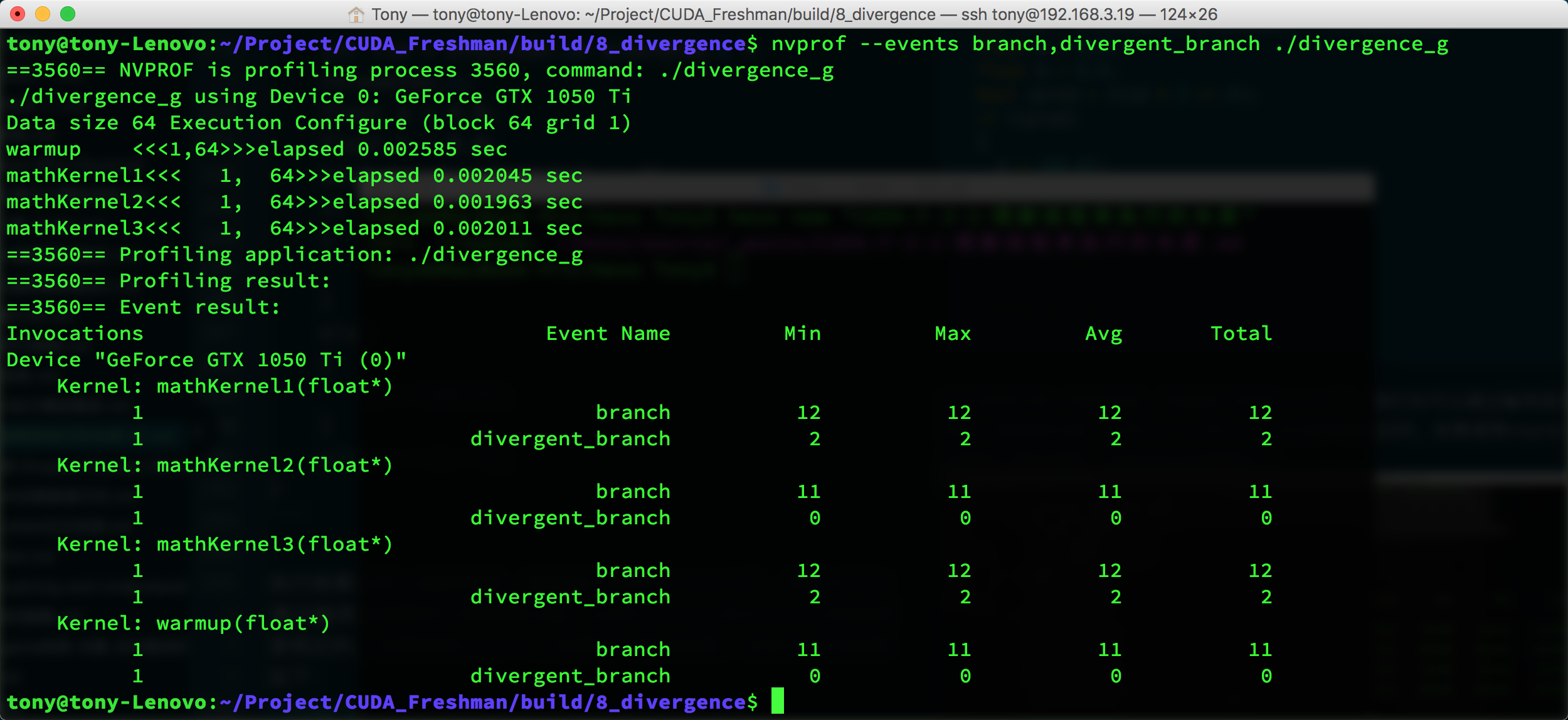Abstract: 本文介绍CUDA执行模型最核心的部分，线程束的执行实质第一部分
Keywords: CUDA分支，线程束分化

# 理解线程束执行的本质(Part I)

## 线程束和线程块$$tid = threadIdx.x+threadIdx.y\times blockDim.x+threadIdx.z\times blockDim.x \times blockDim.y$$

$$\text{WarpsPerBlock}=\text{ceil}\begin{pmatrix}\frac{\text{ThreadsPerBlock}}{\text{warpSize}}\end{pmatrix}$$
ceil函数是向正无穷取整的函数，比如$ceil(\frac{9}{8})=2$

## 线程束分化$$\text{Branch Efficiency}=\frac{\text{Branches − DivergentBranches}}{\text{Branches}}$$nvcc

0%